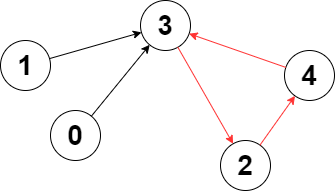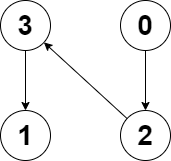## 题目描述

leetcode2360. 图中的最长环```输入：edges = [3,3,4,2,3]

``````输入：edges = [2,-1,3,1]

```

• `n == edges.length`
• `2 <= n <= 105`
• `-1 <= edges[i] < n`
• `edges[i] != i`